Philosophy of Mathematics

Class Notes

PHL-113

Dr. Carl Posy

Duke University

Fall 1992

Prepared by

Part I: General Survey of Philosophy of Mathematics 1

I.1 Prehistory of numbers 1

I.2 Greek Development of Math 2

2.1 Flowering of the Pythagoreans 3

2.2 Downfall of the Pythagoreans 3

2.3 Greek Reaction to the Downfall 4

I.3 Road to Non-Euclidean Geometry 12

3.1 Hilbert's axiomatization of Geometry 12

3.2 The Evaluation of non-Euclidean Geometry 13

I.4 History of the concept of a number 16

I.5 Conceptual Foundations of Mathematics 22

5.0 General Overview of Reactions to Berkeley 23

5.0.1 Kant's Philosophy of Mathematics 24

5.1.1 Introduction of the Notion of a Limit 25

5.1.2 Arithmetization of Mathematics 26

5.2 Cantor 29

5.3.1 Peano 33

5.3.2 Frege 34

I.6 Two of the Three Reactions to the Third Crisis 37

6.1 Platonistic Reaction 37

6.2 Hilbert's Program 40

Part II: Intuitionism, A Third Direction 46

II.1 General Introduction to Intuitionism 46

II.2 Intuitionist's Construction of the Natural Numbers 47

II.3 Intuitionist's Construction of the Real Numbers 47

II.4 Choice Sequences 48

II.5 General view of Brouwer's Intuitionism vs. Hilbert's Formalism 51

Part I: General Survey of Philosophy of Mathematics

Philosophy of Mathematics involves the epistemology, ontology, and methodology of mathematics. Certain aspects unique to mathematics cause its philosophy to be of particular interest:

1) abstractness - math involves abstract concepts

2) applicability - math is used by other sciences such as Physics

3) infinity - peculiar notion specific to pure math, yet a central concept to applied calculations

Specific events caused the evolution of mathematical views in an attempt to eliminate cracks in the foundation of mathematics. The most important of these was the discovery of inconsistencies, or paradoxes, in the foundations of mathematics. This represents the starting point of the modern philosophy of mathematics.

Overview of Part I

1. Prehistory of numbers

2. Crisis in Greek Mathematics, the Calculus Crisis, and the 20th Century Crisis

Crises result from problems between numerical and geometrical mathematical models

4. History of the concept of a number

I.1 Prehistory of numbers - origins of the notion of a number

The Farmer and the Crow

A farmer wanted to shoot a crow who was eating his crop. He went out by himself and hid behind a tree waiting for the crow to come out. Unfortunately, the crow saw him hide behind the tree, and wouldn't return until he'd seen the farmer (and his rifle) leave. The farmer then figured he would trick the crow; he and his wife went out and both hid behind the tree. The farmer then sent his wife back inside, in clear view of the crow. Again, the crow wouldn't come out until the farmer left as well-- the crow could apparently tell the difference between one and two people. The farmer then repeated his attempts with three, four, five, six, and seven of his friends. Not until he brought a seventh friend, and sent all seven friends away from the tree did the crow come out of hiding, at which point the farmer promptly shot the crow.

What's the point?

Although the crow could distinguish between one through seven, it seemed as though it couldn't tell the difference between seven and eight individuals. It could "count" as high as seven, since it has six talons. It could remember the number of people by equating each person to a talon, and thinking one, two, three, four, five, six, more. Since both seven and eight people equate to "more" they were indistinguishable to the crow.

Counting

Counting rests on a 1-1 correspondence; a mapping between the elements of two sets; for example, stones(1) used to count sheep. the set of talons, however, was exhausted with the seventh person, since there were no more talons to correspond to the last person.

Usually the correspondence is set up because we are concerned about the size of one set against the index set.

Numbers are a canonical (universally-accepted) index set.

English numbers are infinite, based on the number 10 (decimal), while French numbers are based on 20.(2)

Babylonians used base 60,(3) which is why time and the circle are divided into multiples of 60. They also altered their number system to simplify calculations within different applications.

There is an important distinction between counting and comparing relative size. Cardinal numbers tell about size, while ordinal numbers are used for counting.

For counting, the order is significant, while cardinal numbers' order is irrelevant.

In English, we make a semantic distinction between the two types of numbers: fifth is an ordinal number, expressing an ordered position, while five is a cardinal number expressing a magnitude.

I.2 Greek Development of Math

Thales is credited with the beginning of Greek mathematics; he gave math the position of a separate science-- it's own area of discourse.

2.1 Flowering of the Pythagoreans(4)

Pythagorean ideas dominated mathematical thinking for a while. The pythagoreans:

represented a coherent body of mathematical doctrines

believed "number rules the universe" - made no distinction between math, physics, or different areas of mathematics

were primarily concerned with the study of properties of counting numbers

believed all measurements could be expressed in terms of natural numbers, or ratios of natural numbers (called rational numbers)

developed number theory(5) (primes, relative primes, amicable numbers, etc.) with applications (eg. harmonics)

developed geometric theorems and ideas about proportions

insistent that mathematical ideas required proofs

thought numbers had concrete representations as figures of points-- developed figurate numbers (eg. square numbers, triangular numbers, etc.)

Three important pythagorean beliefs

1) Agreed with Babylonian assumption of commensurability-- any geometric measurement will be some rational multiple of the standard unit.

2) Thought that space is ultimately discrete (separable)-- there is nothing between 1 and 2. Everything had to have atomic parts.

3) Believed continuity implied infinite divisibility

2.2 Downfall of the Pythagoreans [Crisis #1]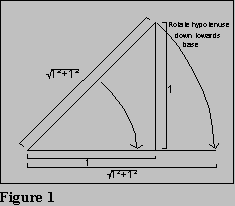a) Commensurability is false (found irrational numbers)

If commensurability were true, (1+1)=2 must equal p/q where p and q are natural numbers.

Proof that Commensurability is False:

1) Assume 2=p/q, p and q {set of natural numbers}

2) Reduce p/q to lowest terms (can be done with any fraction)

3) p=q2 p2=2q2

4) Thus, p2 is an even number (it is equal to twice an integer)

5) p2 = p is also even (all even sqaures' roots are even)

6) So, p=2k, k {set of natural numbers}

7) (2k)2=2q2 4k2=2q2 2k2=q2

8) Thus, q2 is even, so q is even

9) Both q and p are even, thus they share the common factor of 2; this however, contradicts step #2, that p/q is reduced to lowest terms, thus our assumption that 2=p/q is false.

First recorded use of reductio ad absurdum(6) proof

b) Infinite divisibility and discreteness seem inconsistent

Zeno's paradoxes of motion (4 main paradoxes) attack philosophical theories of motion which parallel a pythagorean view of reality.

The paradox of the arrow-- it is impossible for an arrow to move

at any instant of time, the arrow travels over no space, thus it is stationary

if an interval of time is a continuum of instants, the arrow doesn't move during any of the instants, thus it doesn't move during the entire interval either.

Thus, the Pythagorean world view was internally inconsistent.

2.3 Greek Reaction to the Downfall

a) Plato - isolate and defeat the problem

Stressed separation of geometry and number as two separate branches of mathematics, thus isolating math from the study of the physical world altogether.

Thought mathematics belonged to realm of ideas and forms; believed in abstract notions without physical counterparts-- those abstract things comprise a mathematical reality.

Thought abstract reality of mathematics is completely precise and structured-- Abstract is real; concrete is illusory.

b) Aristotle

Gives much more precise account of nature of collections, manifolds

Gives much more precise account of nature of continuity, discrete

1) Aristotle's definition of continuity

Things are together in place when they're in one primary place; they are apart when they are in different places. Things are in contact when their edges are in the same place. A thing is in succession when it is after something with nothing similar (of the same kind) in between.

Contiguous succession is succession along with contact.

Continuous is what is contiguous when the extremities are one and the same, contained in one another. Continuous implies shared, merging edges.

Isomorphisms-- all basic continuous manifolds (i.e. space, time, motion) are isomorphic.

Flowing motion is the basic idea of Aristotle's continuity-- very different from the Pythagoreans' view.

Aristotle pointed out that the problem in Zeno's paradox is due to infinity.

2) Aristotle's analysis of infinity

To get a point requires infinite divisions of a line; anything short of infinite divisions is a continuous magnitude. Infinite divisions is not possible, thus we can't get to a point from a line.

A completed infinity was an incomprehensible, impermissible notion to Aristotle, while a potential infinity is permitted. The idea of a completed infinity was based on an existential claim-- there would exist a point; a potential infinity, instead, contains no existential claim-- any finite number of divisions can be made to a line without exhausting the magnitude(7).

3) Aristotle believed proof methods may by usable only in different contexts. He thought patterns of reasoning should be discussed and science could be organized according to acceptable patterns of reasoning.

Aristotle invented logic. In fact, his basic categorization for logic defined its study until the late 19th century.

His basic idea was that reasoning always requires a starting point. Aristotle believed science is made up of statements, and science is basically about inferring conclusions from propositions. Aristotle showed what inferences were valid and what were fallacious. He analyzed the structure of statements(8), the components of inferences.

Aristotle also introduced the idea of deduction-- that one can infer new statements by building upon accepted past truths. This technique has the problem of infinite regress,(9) hence he developed postulates or axioms to serve as a foundation. Postulates (and axioms(10)) are accepted as true, without requiring proof. All other statements about a science must then be proven to be true using only the postulates, axioms, and previously-proven-true statements.

Aristotle thought that concepts required definition. Again, a hierarchy existed where there were concepts defined as specializations of more broad concepts. For example a square could be defined as a rectangle with four equilateral sides; in this example, the square is the species of the more broad genus, rectangle. Again, infinite regress is a problem, necessitating fundamental concepts which aren't defined (eg. a geometric point). Other concepts are then constructions (combinations, specializations, etc.) of the fundamental concepts.

However, just defining a concept wasn't enough for Aristotle. He required that one must also show an instantiation of the new concept exists. Thus each fundamental (undefined) concept requires an existence postulate (since their existence can't be proven).

c) Mathematicians reaction to the Downfall (Eudoxes and Euclid)

Eudoxes

Theory of proportion:

Four magnitudes are said to be in the same ratio, the first to the second and the third to the fourth, when, if any equimultiples whatever be taken of the first and third and any equimultiples whatever be taken of the second and fourth, the former equimultiples alike exceed, are alike equal to, or are alike less than the latter equimultiples.(11)

So, if x and y are the same kind of magnitude, and z and w are also the same kind of magnitude (not necessarily the same kind as x and y, though), then:

x:y = z:w if and only if:

mx > ny implies mz > nw, and

mx < ny implies mz < nw, and

mx = ny implies mz = nw,

for all m and n.

Note that Eudoxes's theory of proportion is non-numerical; it only describes a basic notion of proportionality.

We can prove that the areas of triangles of equal heights is proportional to the lengths of their bases. In other words, given ABC and ADE, we can show that Area of ABC : Area of ADE = BC : DE(12).

Proof: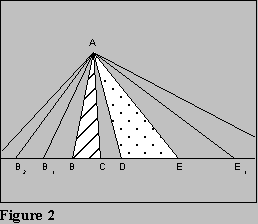Given m and n, we must show:

m × AABC > n × AADE

m × BC > n × DE, and

m × AABC < n × AADE

m × BC < n × DE, and

m × AABC = n × AADE

m × BC = n × DE.

1) Replicate segment BC to the left of B n-1 times; replicate segment DE to the right of E m-1 times, see figure 2.

2) Thus, BnC = n(BC); DEm = m(DE).

3) So, AABnC = n(AABC); and

4) There is a theorem that says if two triangle's share the same altitude, whichever has the larger base has the larger area (likewise for smaller, and equal). Thus:

If m × BC > n × DE, then

m × AABC > n × AADE, and

if m × BC < n × DE, then

m × AABC < n × AADE, and

if m × BC = n × DE, then

m × AABC = n × AADE.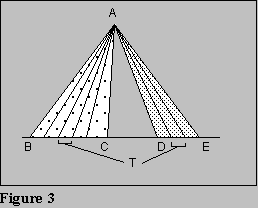INCORRECT Proof:

1) Choose T such that BC = T×P, and DE = T×Q, see figure 3.

2) Divide ABC into P triangles each of base T, ADE into Q triangles each of base T.

3) Each smaller triangle has equal area, so total areas are in proportion.

ERROR: This proof assumes the existence of T, which is false. Thus the proof is unsound-- it is based on a false assumption.

Method of Exhaustion, Basic Assumption:

If from any magnitude there be subtracted a part not less than its half, from the remainder another part not less that its half, and so on, there will at length remain a magnitude less than any preassigned magnitude of the same kind.Proof:

1) Given two circles, A1 and A2 (denoting area), with diameters d1 and d2, respectively, we know A1 : A2 = d12 : d22 (area is proportional to square of diameter), see figure 4.

2) Take circle A1 and inscribe a regular polygon and repeatedly double the number of sides in the inscribed polygon. See figure 5.

3) Given any small , we can eventually get an inscribed polygon, P1 with enough sides such that:

Acircle #1 - Apolygon < .

4) Suppose:

A1 : A2 > d12 : d22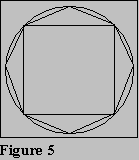Choose Pn such that the inequality holds and thus:

P1 : A2 > d12 : d22

5) Now take P2 to be a similar polygon inscribed in A2.

6) From theory of proportions, we have:

P1 : P2 = d12 : d22

7) Thus, we have:

P1 : A2 = P1 : P2

which implies P2 > A2. However, P2 is inscribed in A2 and thus cannot have a greater area. Therefore, our supposition in #4, above, is false.

8) Through parallel reasoning, we can show that:

A1 : A2 < d12 : d22

is also false, reducing to a similar absurdity.

9) If both inequalities are false, then the only other possible relationship is equality, so:

A1 : A2 = d12 : d22

must be true.

Similar double use of reductio ad absurdum method characterizes almost every geometric use of method of exhaustion.

Resistance to the theorem due to the double use of reductio ad absurdum for positive results.

Very sterile method; the relationship to be proved must be discovered in another way, mathematics is simply used to prove the other possibilities to be false.

Split mathematicians into empirical scientists and theorematic mathematicians.

Archimedes objected to the method of exhaustion and replaced it with the method of equilibrium.

Idea of infinite was very little strips that could be measured on a scale

Archimedes used the method of equilibria for discoveries, but used method of exhaustion for rigorous proofs.

Euclid

Axiomatized geometry into a perfect Aristotelian science (in The Elements). Few proofs are attributed to Euclid, his achievement was the organization: what needs to be a postulate? etc.

Believed in absolute separation of discrete mathematics and magnitudes.(14) For example, Books 5 and 6 state the theory of proportion for magnitudes, while Book 7 states the theory of proportion for numbers.

Thomas Heath wrote a commentary on The Elements (1926). He wondered why Euclid didn't use numbers as a special case of magnitudes and save all the repetition.

Mathematicians made an ontological change after Zeno's paradox-- separation of numbers and magnitudes was commonplace, thus it probably didn't occur to Euclid that numbers (numerical entities) were a special case of magnitudes (geometric entities)

Elements is full of difficulties

1. Absolute separation resulted in a great deal of repetition

2. There were actual gaps

3. There were perceived gaps

Archimedean Postulate (Unstated assumption in the method of exhaustion):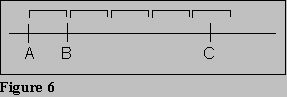If we continuously mark off segment AB in the direction of C, eventually we'll pass C.

This can not be proven from the other axioms.

Gaps were often due to the dependence on pictorial diagrams. Assumptions seemed obvious, but needed to be explicitly stated. For example, Euclid forgot:

Given a line, there must be two points on it, and

Given a line, there exists at least one point not on the line

Euclid should have stated the "self-evident" assumptions.

Perceived overstatement: The Fifth Postulate of Geometry (Parallel Postulate)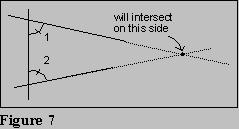If a straight line falling on two straight lines makes the interior angles on the same side less than two right angles, then the two straight lines, if produced indefinitely, will meet on that side on which are the angles less than two right angles. (see figure 7).

Mathematicians objected-- the postulate was too complex, and seemed derivable from the other postulates. However, Euclid was right!

3.1 Hilbert's axiomatization of Geometry

David Hilbert wrote Hilbert's Foundation of Geometry-- a new axiomatization of geometry with about thirty postulates, divided into five categories:

a) Incidence (intersection)

b) Betweenness

c) Congruence

d) Continuity

e) Parallelism

Hilbert points out that the interpretation of terms is irrelevant, only deductive logic is used to generate theorems.

Dedekind's Postulate

Given line l1, split it into c1 and c2 such that l = c1 c2, and c1 and c2 are not empty nor do they equal l. No point of c1 is between two points of c2, and no point of c2 is between two points of c1. Then there is a unique point O such that O is between x and y if and only if x c1, y c2

3.2 The Evaluation of non-Euclidean Geometry

How did Hilbert realize the necessity of the parallel postulate? Many attempts were made to prove the parallel postulate as a theorem, using only the other four postulates.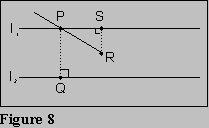Proclus's Proof Attempt:

As PR increases, so does SR. Thus, somewhere, SR PQ as PR is extended (see figure 8).

Can be proven, but requires the parallel postulate!!

John Wallis (1616-1703) - looked for simpler postulates to assume, and tried proving the parallel postulate from those.

Simpler Postulate: Given a ABC and any line segment DE, there exists a triangle with DE as a side which is similar to ABC

In other words, similarity preserves shape-- size, shape, and location, are independent of each other.

This simpler postulate allows derivation of Euclid's fifth postulate.(15)

Saccheri (Italian, 1663-1733) and Lambert (German, 1728-1777)

Saccheri and Lambert independently tried proving the parallel postulate by the reductio ad absurdum method. Their proof started with "neutral geometry"-- Euclidean geometry excluding the parallel postulate.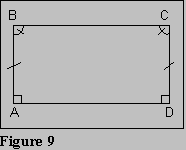Assume B = C, three possibilities exist:

1) B = C = 90

2) B, C > 90 (obtuse angle hypothesis)

3) B, C < 90 (acute angle hypothesis)

#1 is equivalent to the parallel postulate.

#2 can be shown to be contradictory to neutral geometry.

#3 couldn't be proven contradictory; it generated weird results-- "results [that] were repugnant to the nature of the straight line and space."

This was the discovery of non-Euclidean geometry (but they didn't know it)

In the late 18th and early 19th century, three men became interested in the acute angle hypothesis. Bolyai (Polish), Gauss (German), and Zobachevsky (Russian) all took the negation of the parallel postulate as a postulate and added it to neutral geometry. Nothing contradictory followed!

The Negation of the Parallel Postulate:

There is at least one line l and one point p outside of l such that through p there are at least two lines which do not intersect l.

As it turns out, this implies through any point outside of l, there are infinitely many lines parallel to l.

Some theorems that are derivable from neutral geometry and the negation of the parallel postulate:

Angles in a triangles sum to less than 180 degrees.

Angles in a quadrilateral sum to less than 360 degrees.

Rectangles do not exist.

If two triangles are similar, then they are congruent-- size and shape are not independent.(16)

This new geometry is called "hyperbolic geometry."

Riemann developed "elliptical geometry" where there are no parallel lines; all lines intersect.

Klein and Belttrami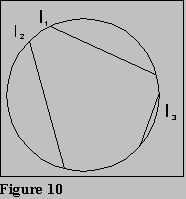independently proved that there is no hope of contradiction between neutral geometry and the negation of the parallel postulate

showed if straight Euclidean geometry is consistent, it must also be true that no contradiction can occur in non-Euclidean geometry.

showed one could model non-Euclidean geometry inside Euclidean geometry.

See figure 10: circle is whole plane, a chord is a line. Notice that infinitely many chords (lines) don't intersect l and are thus parallel.

Translation between notions of normal geometry and interpretation in the hyperbolic plane:

Euclid Hyperbolic

point point interior to circle

line chord of circle

Given the translation equivalence, theorems and postulates can be proven.

These facts inspired Hilbert's remark that a study of geometry is just a consequence of certain axioms and their interpretations are unimportant.

Klein said geometry is really the study of Algebra: a collection of transformations and what remains the same. In fact, geometries can be classified by which properties of figures remain invariant under which transformations; Euclidean geometry is characterized by a certain set of invariants.

Bottom line:

There are several ways to consistently extend Euclid's first four postulates (neutral geometry) in incompatible directions.

Philosophical Questions:

What do the paths have in common?

What is space? What are its properties?

Hilbert believed geometry was just a formal system and the consequences of postulates and axioms studied independently of their interpretations (an uninterpreted formal system). A consistent interpretation of a system (called a model could be given to show consistency.

What makes a sentence true?

Which geometry is the "true geometry"?

The one physics verifies? Why does one work for physics? Why should any work for physics?

An empirical question?

What happens to the other geometries?

Other notion of space?

Meaningless game?

I.4 History of the concept of a number

Respect for Aristotelian idea that a completed infinity does not belong in mathematics.

Need to introduce objects which are infinite in an essential way(17)

Pressure not to admit irrationals as numbers due to their lack of a decimal expansion.

Conflict: separation of magnitude and number, versus applications requiring them to be together. Conflicts arose due to the need to apply mathematics to physical situations.

Nicholas of Cusa - cleric, write, philosopher, and theologian of the 15th century

Liked infinity in theology, so liked it in mathematics also; wanted infinity to be brought back into math

Thought triangle was the polygon with the fewest sides; circle was the polygon with the most sides (infinitely many sides)

"the infinite is that which cannot be made bigger. . . .the infinitesimal is that which cannot be made smaller."

Valerio and Stevin - applied mathematicians

Stevin was interested in the problem of calculating the fluid pressure on a vertical damn. He assumed the damn is made up of thin rectangles and used known formulas for the relationship between the area and volume of the polygons, then rotated the thin strips through the area of the damn.

Valerio developed similar applied methods using thin strips.

After formulas were derived, they were treated as if they were delivered independently of mathematics

Next step? Use this way of thinking inside mathematics.

Cavalieri - introduced a thin strip, or "indivisible" into mathematics. Plane strip can be thought of as infinitely many parallel indivisibles, etc.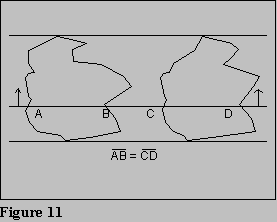Cavalieri's principle:

One can move indivisibles composing a figure independently of each other and thus recreate the figure. Then we have two figures inside two parallel lines. If all lines parallel to two containing lines intersecting the two figures cut chords of equal lengths, then the areas of the two figures are the same.

Cavalieri admitted that his methods clearly couldn't be rigorous; "Rigor is for philosophers, mathematics is for scientists."

Cavalieri's chief critic was Galileo.(18) Galileo launched another attack against the notion of infinity:

Let's think about a set of all natural numbers. It is infinite. This set is clearly larger than the set of all perfect squares. However, there is a one to one correspondence between the set of all natural numbers and the set of all perfect squares. Thus the sets have the same size. A paradox is apparent.

Thus, mathematical notions don't apply to infinity; we can't speak of larger or smaller than infinity. Infinity is not a magnitude, it is a collection of aggregates. Thus Galileo didn't permit Cavalieri's method in mathematics, but did teach his method to students, and advised them to use it to solve problems.

Pressure moving towards the notion of integration, yet still viewed as non-rigorous.

Theorem

If two solids have equal altitudes and if sections made by planes parallel to the bases and of equal distance are always in a given ratio, then the solids' volumes are also in that ratio.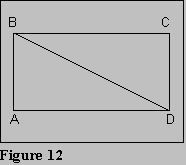See figure 12:

Area DBC : Area Rectangle = 1:2

Now build three dimensional figure out into space from whole rectangle and for triangle (forming a half-pyramid)

Area Half Pyramid : Area Parallelopiped = 1:3.

Note that these observations using modern calculus notation are: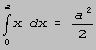and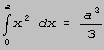These generalize to the well-known integration formula: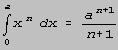This generalization came much later; for the individual results, each calculation's methods were different. It was only conjectured that the pattern must hold in general. The notation itself wasn't understood.

Heterogenia is the notion that geometric figures are made of lots of figures in lower dimensions. The above methods use the idea of summing these figures of lower dimensions to make calculations about the figures of the higher dimensions

Heterogenia was a very controversial idea!

1) infinitary methods allowable in mathematics and/or usable for calculation?

Cavalieri didn't mind because his idea of mathematics didn't require rigor.

Galileo didn't accept the methods into math, but used for calculations

2) infinitesimals vs. indivisibles

Infinitesimals are objects which make up a figure; their size decreases as their number increases.

Indivisibles are the basic, atomic components of a geometric figure.(19)

Keppler gave a proof for the area of the circle by summing the areas of infinitely many small triangles with heights equal to the circle's radius:(20)

h=r, sum of base length = circumference of circle = 2r, thus,

area of circle = ½(2r)r = r2.

3) geometric vs. numerical methods

Cavalieri thought everything should be viewed geometrically.

Vieta instead worked with algebraic notions; he represented variable magnitudes by letters, and described geometric figures by equations that would lead to their graph. This analytic geometry was rejected by Cavalieri because in an equation typical of analytic geometry such as x3 + x2 + x = 0, terms corresponding (geometrically) to a volume, area, and magnitude were being added-- this summation of unlike terms was abhorrent to Cavalieri.

John Wallis, a mathematician in favor of the idea of the indivisible, and in favor of numerical methods attacked the same parallelogram problem as previously mentioned. He introduced to represent the largest number. Thus, according to Wallis: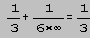since the reciprocal of the largest number must be the smallest number.

Wallis also conjectured the integration formula:Also calculated the area of a triangle to be ½bh by summing infinite trapezoids.

Wallis's proofs used properties of numerical progressions.

Isaac Barrow(21) also rejected analytic geometry. He favored geometrical methods and the concept of the infinitesimal. Barrow was concerned with determining tangents to curves, but wouldn't view curves as equations.Take an indefinitely small arc MN, and construct MNR, and compare NR:MR to NP:TP. There are several rules Barrow followed when considering the idea of M moving closer and closer to coincidence:

1) Omit all terms containing e or f to a power greater than one.(22)

2) Balance

3) Assume as M approaches N, the lines coincide.

Invention of the Calculus by Newton and Leibniz

rivalry developed

colorful personalities

interesting story-- Who was first? Any plagiarism?

Newton, born in 1642, had written ideas about the Calculus in the 1660s, but Leibniz, born in 1646, had already published similar ideas. What makes things more complicated is that there was a good deal of correspondence between the two of them.

No clarification whatsoever of the confusion in mathematics!

Newton's and Leibniz's contributions were formal, technical improvements; they systematized and generalized the confusion of methods. They did not have any better conceptual methods than others. From the promulgation of their methods, the Calculus was immediately thought of as a new branch of mathematics. Today, we use Newton's reasoning, and Leibniz's notation.

Newton dealt with the analysis of motion; he viewed curves as the locus of motion of a point and believed that notions of motion and flow must be used when analyzing continua. He called his discovery the method of fluxions. Curve was a mapping between abscissa and ordinates. Variables were called "fluents"; rates of change were called "fluxions." The moment of a fluent was the delta of a variable. Newton's notation was as follows:

x is a fluent;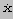is a fluxion;(23) o represents a small t

Notation of lowercase "o" was misleading-- it looks like zero, and people accused him of letting the t equate to zero.

Important contribution was recognizing the existence of an abstract technique which was applicable to all problems involving rates of change.

Leibniz's notation:. dy and dx are both very small that they are insignificant, however, their ratio is a number; thus ratios were stressed, not the individual components.

Impact of Newton and Leibniz was due to systematicity and applicability-- not at all a conceptual improvement. There was no longer confusion, math had a new science: infinitesimal analysis; it was so powerful that the face of mathematics was changed forever. It was called the Second Great Crisis because math is proceeding on the basis of conceptually unclear notions; Physics was being based on these questionable ideas. These problems brought serious attacks against mathematics.

George Berkeley criticized the whole process of the Calculus (in The Analyst, 1734)

very effective argument that the Calculus is an unacceptable scientific practice.

objected to the notion of instantaneous velocity.

tried recreating Zeno's paradoxes in Newtonian Calculus.

thought the notion of instantaneous velocity has no physical velocity

shows there is no consistent mathematical position on the issues.

saw math as the science of extended things; infinitesimals don't have extensions.

mathematical techniques don't mesh with the reality of the times

Reaction to the challenge was opposite to the Greek's earlier reaction:

Keep mathematics, keep the Calculus; dig in and discover a clear conceptual foundation!

I.5 Conceptual Foundations of Mathematics

Infinite quantities are not picturable-- maybe that's okay. So then what is mathematics about?

What are adequate grounds for mathematical reasoning if not picturability?

1) Math is just an abstract science with strict definitions; it is simply a matter of proof and rigor.

2) Math is about the physical world but we have to learn how to use the appropriate theory about what we perceive-- we need a theory of intuition to allow us to keep the infinitary parts of mathematics.

Within math, it was recognized that the problems arise because of the unclarity that the mathematicians had about the relationship between geometric methods and numerical methods. Geometric methods which allowed the infinitesimally small are too imprecise. This led to the introduction of arithmetic techniques to the study of infinitesimal analysis(24) to give it rigor-- a return to Pythagorean ideas.

5.0 General Overview of Reactions to Berkeley

Two Philosophical Lines

1) Math should be limited by the nature of perceptual faculties; it should be intuitive, and should be about the perceivable world. Hence, what is needed is a more sophisticated theory of perception.

2) Problems in math are not consistent with perceptual abilities, but do not limit math to what is intuitable; math isn't necessarily about perceivable things, it should be governed only by abstract considerations of rigor and reasoning.

The first reaction within the philosophical community was the first idea above. Imanual Kant(25) and John Stuart Mill(26) took this view.

On the other hand, the mathematics world supported the second philosophical line. This was called the anti-Kantian direction, as it opposed Kant's views. The reason for this direction was related to two developments in pure math:

Consistent alternative theories of Geometry showed that mathematics can be presented as an abstract system without any particular interpretation.

Arithmetization of mathematics proved math could be reduced to simply the concepts of natural numbers and sets.

There was still resistance to leaving intuition behind. Kronecker,(27) for example, advocated a return to an intuitive basis of mathematics.

Another Crisis: Abstract non-intuitive mathematics was internally inconsistent!!!

5.0.1 Kant's Philosophy of Mathematics

First Thesis:

Mathematics is about the empirical world, but it is special in one important way: necessary(28) properties of the world are found through mathematical proofs; to prove something is wrong, one must show only that the world could be different.

Epistemological Problem:

Sciences are basically generalizations from experience, but this can provide only contingent, possible properties of the world (it could have been otherwise). Science simply predicts that the future will mirror the past.

Mathematics is about the empirical world, but usually methods for deriving knowledge give contingent knowledge, not the necessity that pure mathematics(29) gives us. Kant wants necessary knowledge with empirical knowledge.

Kant Solves the Problem in a Couple of Steps

1) Objects in the empirical world are appearances (or phenomenon). By their nature, they have only the properties that we come to know of them from experiences. They are not things in themselves. Therefore Kant said we must:

Become an idealist-- object's properties are only what is perceivable; there are no non-experienceable properties of objects.

2) Built into our minds are two forms of intuition and perception such that every perception we have is shaped by these forms: Space and Time. These are, in fact, parts of the mind, and not something the mind picks up from experience. Thus, empirical objects are necessarily spaciotemporal objects.

3) We then come to know spaciotemporal properties in an a priori fashion; in studying spaciotemporal properties, we are merely studying ourselves, and our perceptual abilities.

4) Mathematics is simply the science that studies the spaciotemporal properties of objects by studying the nature of space and time.(30)

Thus, mathematics is the studying of the abstract form of perception.

What about infinitary ideas-- things not subject to perception?

Kant makes a distinction between:

Empirical intuition, the intuition from the senses which is always finite (mathematics doesn't deal with this), and

Pure intuition, the study of possibilities for empirical intuition where finite limits are not introduced in either direction.

Thus mathematics can allow the division of small intervals and the expansion of large intervals. This means we can discuss smaller and smaller quantities without introducing the smallest quantities.

Suppose we want to prove an interval is divisible. We can do this by the following:

1) Pick an interval

2) Show it is divisible

3) Abstract from its actual size, and let it represent the notion of a perceivable interval.

Two Consequences of Kantian View

No such thing as unapplied mathematics-- math is, by nature, about the world (if it's not, it's just an abstract game).

There is exactly one right mathematical theory of time, space, and motion.(31)

5.1.1 Introduction of the Notion of a Limit

Instead of talking about infinitely small quantities, think of a sequence of smaller and smaller quantities approaching a number. This idea was formulated independently by Bolzano and Cauchy in the late 18th century.

Bolzano's Definition of a Limit

When F1(x), F2(x), ..., Fn(x), ... is such that for any given small quantity the difference between Fn(x) and Fn+r(x) gets and stays smaller than that quantity as n gets larger, then there is one and only one magnitude to which the sequence gets as close as you please.

Today, this definition is considered an example of an internal criterion for convergence.

Cauchy's Definition of a Limit

When the successive values attributed to a variable approach indefinitely a fixed value so as to end by differing from it as little as one wishes, this last is called the limit of all the others.

Difference between definitions: Cauchy's talks about a relationship to the end! He gives an external criterion for convergence.(32)

5.1.2 Arithmetization of Mathematics

First step was the precise notion of what a limit is; Bolzano and Cauchy took care of that.

Applications of converging to a limit:

Cauchy used limits in describing the notion of a derivative. He also introduced the notion f(x):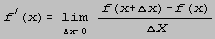This used an Aristotelian potential infinity, instead of an actual infinity.

Application to the notion of a continuous magnitude:

When is a line (or function) continuous?

A function is continuous at x0 if as xx0, f(x)f(x0). The function (as a whole) is continuous if it is continuous for all points x0.

Thus, the notion of continuity was made into an arithmetic notion (from a geometric notion).

Cauchy tried using the limit notion for defining, and actually building, irrational numbers out of limits and series. He said that irrational numbers are the limit of a sequence of rational numbers. For example: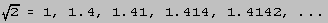(defined as limit of the series)

Cauchy was right thatshould be viewed as a number, but his attempt to show the convergence failed because of his definition of a limit. He defined an irrational number as the convergence of a sequence, but his definition of a limit required knowing the limit in advance (in order to get closer and closer).

Cauchy assumed internal convergence established the existence of a limit; he was missing the step of defining what sort of entity the limit of a sequence is. Karl Weierstrass(33) added the missing ingredient:

The problem with Cauchy's definition is there is no entity for the limit of the sequence to be. Let the limit of a convergent series of rational numbers be the set of numbers in the series. Therefore a set is a number. Hence, the limit of:

1, 1.4, 1.41, 1.414, 1.4142, ...

is the set:

{1, 1.4, 1.41, 1.414, 1.4142, ... }

and: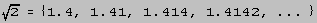Weierstrass also introduced the - definition of a limit: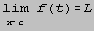if for all > 0, there exists a > 0 such that for all t: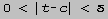implies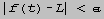We are now back to the Pythagorean idea of a single theory based on numerical concepts.

Weirstrass' definition of an irrational depended on it being a series; the notion of continuity reduced to the idea of a sequence of rational numbers and convergence.

Richard Dedekind took it one step more abstract. He showed the definition of irrational number can be made only with the notion of sets of rational numbers (eliminating the need for notions of series or convergence). Suppose we have a set of rational numbers and cut the set into parts A and B, exhausting the set. All rational numbers are in either set A or set B, none are in both, and all elements of A are less than all elements of B. We can then define the abstract notion of a real number as a pair, {A,B} of sets. For example: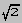is defined by sets {A,B}, such that:

A is the set of all rational numbers x, with x2 < 2, and

B is the set of all rational numbers y, with y2 > 2.

Dedekind's view is more abstract, but requires less conceptual machinery-- irrationals are defined in terms of only rationals and sets.

This view signifies a retreat on one crucial issue: Actually infinite sets must be legitimate objects.

George Cantor showed a consistent theory of infinite magnitudes exists. He defined natural numbers in terms of sets.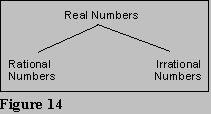Frege showed nothing else but an infinite set is required; he thought of math as a branch of pure abstract logic.

Mathematics is built from classification of numbers shown in figure 14. Each level has its own set of defined operations: N, the natural numbers, have operations defined. Q, the rational numbers, are defined as ratios of N and their operations are defined in terms of operations on N. R, the real numbers, are defined as sets of convergent sequences of rational numbers or as Dedekind cuts

It is important that:

There is a subset of Q isomorphic to N, and

There is a subset of R isomorphic to Q.

The operations must be defined to preserve these isomorphisms.

The number one viewed as an element of N is a different entity from the number one viewed as an element of Q is a different entity from the number one viewed as an element of R.

So, ontologically, basic objects of mathematics are N (the natural numbers) and sets! Two developments that this leads to are:

1) Investigation of the notion of infinite sets as numbers and that an infinite set can be a number.

2) Investigation of the nature of natural numbers.

5.2 Cantor

Cantor wasn't concerned with what a number is. Instead, he wondered:

When is it that two sets of objects have the same number?

Cantor, therefore, defined the notion of similarity of size (i.e. equality of cardinal):

Two sets have the same cardinality if there exists a one to one mapping between them which exhausts them both.(34)

Similarly, set A has cardinality cardinality of set B if there exists a one to one mapping from set B to set A which exhausts B.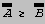Take E, the set of even numbers, and N, the set of natural numbers: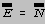yet E N, so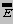should be greater than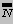.(35)

Dedekind said an infinite set is a set which can be put into a one-one correspondence with a proper subset of itself.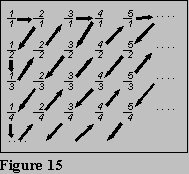Cantor showed cardinality of Q = cardinality of N by showing one to one mapping.

Proof: Write out rational numbers as shown in figure 15. All rational numbers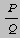appear on the grid. Now give them the order shown by arrows. This orders the rational numbers, thus creating a one to one correspondence with N. The first rational number in the ordering corresponds to one, the second corresponds to two, etc.

A denumerable set (sometimes called enumerable) is one that can be put into a one to one correspondence with the set of natural numbers.

Cantor conjectured that there are only two types of cardinal numbers: finite or infinite; thus all infinite sets would be of the same size. However, he proved this conjecture false! The set of R is larger than N; in fact there are more real numbers between zero and one than there are total natural numbers.

Diagonal Proof:(36)

Assume that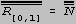. List all natural numbers down left side, and give corresponding real number to each's right:

1 0.a11 a21 a31 a41 a51 a61 .....

2 0.a12 a22 a32 a42 a52 a62 .....

3 0.a13 a23 a33 a43 a53 a63 .....

4 0.a14 a24 a34 a44 a54 a64 .....

5 0.a15 a25 a35 a45 a55 a65 .....

6 0.a16 a26 a36 a46 a56 a66 .....

etc.

List can be completed

Every real number between 0 and 1 occurs in the list

Now select r = 0.d1 d2 d3 d4 d5 .....

Let dn = 3 if ann 3, otherwise let dn = 4.

In other words, let r differ by at least one digit (the diagonal digit) from all the real numbers listed. Thus r is different from each rational at least one digit, so: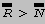because one to one function of N into R exhausts N.

Cantor noticed the proof can be generalized:

Given any set K, the power set of K, P(K) = { S | S \&} K }. The following is then true: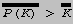Recursively, a set of subsets of a set of subsets is an even larger infinity, and so on. This implies that there exists an infinite hierarchy of larger cardinal numbers. In general:

The power set of any set has a size of greater cardinality.

Cantor's Notation:= 0 (the cardinal number of the natural numbers(37))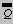= 0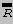=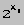Note that this notation is linked to the fact that:

P{1,2,3} = 8 = 2# of elements

The set of function from R to R =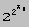Another reason for this notation is that we haven't yet proved that there is no set A such that:(38)

0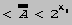Additionally, Cantor showed how to do arithmetic with the infinities:

0 + 0 = {0,2,4,...} {1,3,5,...} = {0,1,2,3,4,...} = 0

02 = 0 0 = 0.

In general, don't operate with the cardinal number itself; instead, do a set-theoretic operation on sets with cardinalities of the given numbers.

Question remains: What type entity is a cardinal number? The distinction between cardinal and ordinal numbers is unimportant with finite numbers because their makeup coincides; however with infinite numbers, a distinction is necessary.

Cantor's notion of ordinality (order-type) was defined as there being an order-preserving one to one correspondence. For example, the set of natural numbers are of the same order-type as the set of even numbers because there exists an order-preserving one to one correspondence between the two sets (i.e. 0 0, 1 2, 2 4, 3 6, etc.).

Thus, N and Q are of different order types! The elements of Q don't have a sequence-- there is no next number. Thus there are two order types within denumerable sets.= , and=

Again, we can perform arithmetic on these order-types:

1 + = because {0} {1,2,3,...} = {0,1,2,3,...} =

However:

+ 1 because {1,2,3,...} {a} = a set with an end having no unique predecessor (different from which has no end).

Thus, the commutative law doesn't hold for ordinal arithmetic.

* is the order type of negative natural numbers, so:

* + 1 = *

In fact, there are 20 different order-types of denumerable sets.

Outcome of Investigation of Infinity:

Infinity is no longer taboo

Infinity is accepted as a notion with rich content, central to mathematics.

A conceptual foundation for the calculus was provided-- all notions of mathematics was reduced to the ideas of natural numbers and the (possibly infinite) set.

No appeal whatsoever to intuition in mathematics anymore.

Resistance from Leopold Kronecker(39)

Kronecker engaged in criticism of Cantor. He thought all Cantor did was nonsense-- just the artificial work of man.(40)

Now mathematics has been reduced to natural numbers and sets. However, the questions remain: Where is the rigor behind natural numbers? What are natural numbers? Why does the reduction stop there? Thus, there is a general move towards creating a non-intuitive conceptual framework for natural numbers.

5.3.1 Peano

First attempt was by Peano.(41) He goes back to Euclid's idea to give a complete axiomatization of the framework. Peano gave five axioms about the notion of a natural number:

1) 0 is a natural number

2) Every natural number, k, has a unique successor, k.

3) If k = m then k = m.

4) 0 is not the successor of any natural number.

5) Axiom of mathematical induction:

If 0 has some property, P, and if n having property P implies that n also has property P, then every number has property P.(42)

This gives a rigorous characterization of the notion of a natural number. However, several problems still exist:

Doesn't tell what a number is; the basic notions (i.e. number, successor, and 0) are left undefined.(43)

Doesn't uniquely characterize the set, N, of natural numbers. Lots of sets fit into the pattern, for example, E, the set of even numbers fits into the pattern as well.(44)

5.3.2 Frege

Frege(45) was motivated by a desire for an ontologically satisfactory notion of a number, but also by a need for rigor. His analysis of the ambiguity of mathematical notion is considered a gem of modern philosophy. Frege believed a language is made up of two kinds of terms: those referring to individual objects (names), and those referring to relationships between objects (predicates). Frege points out that numbers aren't properties of objects.

Frege believed that for mathematics, we should dispense with natural languages and use a formal language instead. He then invented an artificial language with the ambiguities removed.(46)

Frege's account of natural number is ontological-- he says numbers are names referring to certain sorts of sets. This clever choice of the object to which numbers refer reduces all of mathematics to the single notion of a set.

Frege's Principle of Comprehension:

To every property there corresponds a specific object (called the extension of that property) that is the set of all things that have that property.

Notation:

{ X | PX } is the set of all X such that X has property P. For example:

{ X | X X } = ���D\$, the empty set.

Consider M = {Groucho, Chico, Harpo}. Property is being the same size as M. Extension of that property = { X | X is the same size as M } = 3 =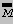.

By this, the number 3 is a set with cardinality 0. The number 0, however, has cardinality of 1.

As always, there are problems:

Only expresses the cardinal aspect of what a number is-- does nothing for ordinality.

Doing arithmetic becomes difficult.

Frege, therefore, took one final step. He recognized that what we needed were related canonical sets. Thus he defined the natural numbers as follows:

0 = The extension of the property:

being the same size as the set { X | X X }.

1 = The extension of the property:

being the same size as the set { X | X = 0 }.

2 = The extension of the property:

being the same size as the set { X | X = 0 or X = 1}.

and so on. Note that this is a recursive definition in which the number n is defined in terms of smaller numbers. This fact gives the natural numbers a sense of ordering (how closely are they related to zero).

Thus, Frege's account of natural numbers is that sets are the fundamental notions out of which numbers are build. Natural numbers depend only on the notions of a property, the extension of a property, equality, and negation-- all of these are notions from logic, not mathematics. Hence, Frege ultimately concluded that mathematics is simply a branch of pure logic. Note Frege's definition is ontological. It is also anti-Kantian in that math is clearly freed from any appeal to intuition. Rigor was achieved through definition and the use of artificial language. Frege supported Platonism: the idea that mathematical objects are abstract to the extreme-- there can be no interaction with mathematical objects through the senses.

Two Types of Properties

A first-order property is a property that applies to objects.

A second-order property is a property that applies to other properties.

Doctrine of Logicism - The view that mathematics is a branch of logic.

Frege's definitions of mathematical objects makes use only of logical ideas: or, not, equals, set, property, and extensions. Thus any being capable of clear thought and understanding the simple concepts has the complete foundation necessary for mathematics.

Frege's work is considered indispensable-- there now is a firm foundation for mathematics!

Bertrand Russell's work brought Frege's work into the public eye.

Problem:

Russell noticed that there existed a difficulty in the basic notion that every property has an extension.

Some properties are self-referential (they apply to themselves). For example, the property of being abstract is self-referential: the set of all things that are abstract includes the set of all abstract things. Thus the set is a member of itself. Conversely, a non-self-referential property has an extension which does not include itself. For example, the property of being a building: the set of all things that are buildings (the set of all buildings) does not include itself.

Frege's assumption was that every abstract notion has an extension. Consider the properties of being a member of oneself and not being a member of oneself. We have: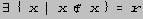, and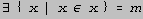.

Let a = {z | z is abstract}, b = {w | w is a building}. Now notice that: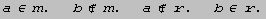More importantly, r is not an element of itself (by definition). We will try to prove this by a reductio ad absurdum method:

Assume: r r. This implies r {x | x x}, so r r. This contradicts with the initial assumption, thus r r. Yet the fact that r is not a member of itself shows r is indeed a member of itself (the set of things which are not members of itself). Thus the existence of r is contradictory.

As we can see, therefore, the principle of comprehension is internally inconsistent. This is very unfortunate! All of math has been reduced to a few simple notions, but now there is an inconsistency in one of those simple notions! Does this mean that all conceptual thought is ultimately internally inconsistent?

This is the Third Crisis. The Crisis in the Foundation of Mathematics.

I.6 Two of the Three Reactions to the Third Crisis

There were three main reactions to the crisis in the foundation of mathematics:

1) Bertrand Russell's Platonism-- maintain Frege's work, just patch up its problems.

2) David Hilbert's Formalism

3) Brouwer's Intuitionism

Both reactions two and three were anti-platonic; they favored recoiling from the abstractness of mathematics, and refounding it on intuition.

6.1 Platonistic Reaction

Alfred North Whitehead and Bertrand Russell collaborated on a work called Principia Mathematica for over a decade. This work:

Carried out the logicists program with a passion.

Demonstrated a construction of the main parts of mathematics going far beyond Frege's work.

Went into detail of the theorems of Number Theory and The Calculus, all from Frege's basic definitions.

Was carried out entirely in an abstract formal language-- a forerunner of modern logic notation.

To remove Russell's paradox from Frege's theory, Russell and Whitehead refined the notion of a set by introducing Type Theory. In type theory, no set can be an element of itself; the thought of a set being an element of itself makes no sense. In type theory, there are different levels of objects:

Level 0 objects are simple objects,

Level 1 objects are all sets of level 0 objects,

Level 2 objects are all sets of level 1 objects,

Level 3 objects are all sets of level 2 objects, and so on.

Thus, everything that exists gets typed at a certain level. It then becomes obvious that nothing could be an element of something of its own level or lower. This, in fact restricts the ability to form sets since each set must be capable of being typed at a certain level. This system results in there being no such thing as a universal set, because it must come in at a certain level, but the level one high up would be larger.

Overall, Type Theory changes the analysis of the notion of a set.

Kurt Gödel's, John VonNeuman's, and Paul Bernaceraf's solutions (independently discovered) created a new set theory, now called GVB set theory. They opposed type theory because it was philosophically and mathematically ad hoc (axioms needed to be added to type theory to make everything follow properly).

GVB Set theory was based on the idea of classes. Every property had a corresponding class, and there were two types of classes: sets, and proper classes. The notion of a set was basically the same as with Russell and Whitehead. Proper classes were very large classes that never came into being at a level; they were never elements of anything (the universal class, for example, is a proper class).

Positive Aspects of the Platonistic Reaction

Gödel proved in 1940 that the continuum hypothesis is consistent with the axioms of set theory; he used the same method as used for Euclid's fifth postulate-- give a nonstandard model and show that all axioms hold, as well as the axiom whose consistency we want to test holds. Gödel constructed an inner model of set theory reinterpreting through a set, L, and showed that all axioms of set theory held, and additionally proved the continuum hypothesis. Gödel also showed that the axiom of choice is true within L.

Axiom of choice gives a new method of making sets. It lets one arbitrarily put objects into a set, thus implying that sets whose elements we can never describe exist. For example, we can construct a countably infinite set of rational numbers, one element from the interval [0,1], one from [1,2], etc.; this set, since it's infinite, can not be listed, and since it's random, cannot be described.

Cohen, in 1962, used an inner model and forcing techniques to show that the negation of the continuum hypothesis was also true. Thus, the continuum hypothesis becomes an undecidable question, like the parallel postulate.

Gödel's work led to set theory's becoming an autonomous, highly-developed branch of mathematics. It also becomes generally accepted that set theory is the foundation of mathematics.

Negative Aspects of the Platonistic Reaction

Set theory is now being challenged as the foundational theory of mathematics. Category theory was proposed as a replacement. In category theory, the basic notions are of function and operation.

Ontological Questions Raised

How accurate is the idea that sets are the basic objects of mathematics?

Set theory is too rich; there are too many different ways to build up the rest of mathematics.

The basic element shouldn't be arbitrarily picked, yet nothing dictates its choice. The modern view is structuralism: the basic units are structures, not actually objects

In the late 1960's, Paul Bernaceraf wrote paper called What the Numbers Could Not Be.(47) Benacerraf claimed that numbers couldn't be a specific thing, because there is nothing forcing them to be anything specific. This leads to structuralism. In structuralism, to be a natural number, is to be a place in the sequence.

If mathematics is totally abstract, why should it have any applicability? Is it just a "miracle" that mathematics applies to the physical world, or, instead, do we tend to emphasize mathematical structures which relate to the world? This is complicated by the numerous new applications for obscure mathematical methods, for example the applicability of group theory to linguistics.

Epistemological Question Raised

How do we know what we know about mathematics without intuition?

Causal theory of knowledge. We know about properties of objects through interacting with them, yet mathematical knowledge lacks a causal chain.

Gödel, in 1964, wrote What is Cantor's Continuum Problem? He said that we do have intuition; not sensory intuition, though. Instead, Gödel believed intellectual mathematical intuition exists and is developed through sufficient practice of mathematics.

6.2 Hilbert's Program(48)

Hilbert had an anti-Kantian reaction now called formalism. Hilbert had two conflicting goals while developing formalism:(49)

Knowing the difficulties of the Platonists' approach, he wanted to move mathematics back to the concrete, recoiling from the abstract. Hilbert wanted an intuitive basis for mathematics, without any appeals to infinitary entities, since there are no such entities in science, the world, or anywhere. Mathematics is just a science, and it has no right to create infinite entities. Hilbert believed the paradoxes serve to illustrate the problems that loosening ties to the perceptual world will result in. Basically, he thought that the only allowable reasoning is finitary reasoning.

But,

Hilbert did not want to lose any part of mathematics. He refused to give up the infinitary methods which worked so well: "No one will expel us from the paradise where Cantor has led us."

The result of these conflicting goals is called Hilbert's Program. The program is implemented in two steps:

1) Divide all of the mathematical sciences into two broad classes: The real part (or conceptual part) of mathematics and the ideal part of mathematics. The real part included only the parts of mathematics which don't take us into the infinitary realm. It involved no ontological or epistemological questions. Logic, and number theory are part of real mathematics.

The ideal part of mathematics included everything else-- all the parts of mathematics that would have been discarded without Hilbert's second motive. This includes geometry, set theory, and analysis.

Hilbert had the basic idea that any branch of mathematics can be formalized (meaning it can be expressed in a formal language(50)) and can be axiomatized to give a formal system.

2) Hilbert observed that a formal system by itself is nothing other than a set of symbols and rules for dealing with them. Symbols and rules belong to the real part of mathematics. Thus, the science of dealing with formal systems (proving properties, etc.) belongs to the real realm of mathematics. Among the properties we should be able to prove is that of consistency. Consistency implies that no contradictions will arise when dealing with the system. The method of proving consistency belongs to the real part of mathematics.

The science of dealing with formal systems is called metamathematics. The usual way to prove consistency is to model the formal system in concrete mathematics and then show that the model was consistent.

Hilbert's program was applied in three steps:

1) Formalize the branch of mathematics to get a formal system, S.

a) Design an appropriate formal language for the branch.

b) axiomatize the theory in that language.

2) Show that the formal system, S, is adequate. In other words, the axioms must really give a formal system for the desired branch of mathematics. There are two things that must be proven to imply adequacy.

a) Soundness. Every theorem derivable from the formal system must be true in the branch of mathematics the formal system implements. No false consequences may follow from the axioms.

b) Completeness. Everything true in a branch of mathematics must be derivable as a theorem from the axioms of the formal system.

3) Prove that S is consistent.

Successes in Hilbert's Program

Hilbert and his student Ackerman produced a formal system for logic.

Hilbert created a formal system for geometry.

Russell and Whitehead's Principia Mathematica formalized number theory.

Two of Hilbert's students, Earnst Zermelo and Abraham Fraenkel, formalized set theory.(51)

Kurt Gödel was a major player in Hilbert's Program. Gödel's dissertation proved the completeness of (first-order) logic. This proof became known as Gödel's Completeness Theorem.

Gödel also proved that Hilbert was right about his assumption that metamathematics was part of the real part of mathematics. Gödel used number theory as a completely concrete example. He then showed how to translate talking about symbols into talking about numbers. He assigned a code to each symbol in such a way that these so-called Gödel-numbers multiplied together represent a formula, sets of formulas, and other things. Then one can talk about the Gödel-numbers using number theory.

Constructing Gödel-numbers:

To make a Gödel-number for a statement in a formal system, you must first assign each symbol a distinct integer starting from one. Then assign each position in the statement consecutive prime numbers (starting with 3). The Gödel-number for the statement is the product of primes taken to the power of number assigned to the symbol in that position of the statement. Since the number two is not a factor of the Gödel-number for a statement, all statements' Gödel-numbers will be odd.

Gödel-numbers for sequences of statements (such as in a proof) are constructed by multiplying out consecutive primes (starting with the number two) taken to the power of the Gödel-number of the statement appearing at that position in the list. For example, if Ai is a statement in a proof and A1 is the first line of the proof and An is the last line, the Gödel-number for the list of statements is:

2g(A1) × 3g(A2) × 5g(A3) × 7g(A4) × 11g(A5) × ... × Png(An)

To say something is a theorem means we can write down a list of sentences which is a proof of it. Thus a theorem's Gödel-number is the last sentence in an even Gödel number. This reduces proofs of theorems to a number-theoretic property involving Gödel-numbers. Thus consistency can be shown through number theory. Gödel showed anything that we can represent in a formal system of number theory is finitary.

Alan Turing(52) defined calculable functions as those that were programmable to be calculated by a simple machine. Those calculable functions are the same as what Gödel came up with. In fact, all differing definitions of calculable functions gave the same set of functions. Notice that, at most, there are 0 calculable functions because are at most 0 ways to program a Turing machine. The number of functions possible are 20, so calculable functions are (theoretically) a rare exception.

Incalculable functions are those whose output depends on a random variable. Consider a function defined to equal one if there is a run of seven 7s in the decimal expansion of the number , and equals two if there isn't. This function is indeterminably calculable; it is calculable if a run of seven 7s is found, but as the decimal expansion continues to be generated, if there still hasn't been a run of seven 7s, we're unable to calculate the function.

Problem

Gödel showed Hilbert's Program can not succeed. This was proven in what is now called Gödel's Incompleteness Theorem. This theorem states:

Let S be a formal system for number theory.

If S is consistent, then there is a sentence, G, such that neither G nor the negation of G (written G) is a theorem of S.

Thus, any formal system sufficient to express the theorems of number theory has to be incomplete.

Proof:

S can prove P(n) just in case n is the Gödel-number of a theorem of S. There exists k, such that k is a Gödel-number of the formula P(k)=G. This statement says of itself, it is not provable.

Even if we define a new formal system S = S + G (thus including the undecidable theorem as an axiom), we can find G which isn't provable in (is independent of) S.

The reasoning Gödel used for his incompleteness theorem is finitary, so it could be formalized inside S. Thus, S can prove that if S is consistent, then G is not provable. Note that the underlined phrase is what G says, so S proves Cst(S)(53) implies G is true, but G says G is not provable.

Suppose S can prove Cst(S), then S can prove G, but if S is consistent, it can't prove G, thus it can't prove its consistency. Thus, Hilbert's Program does not work; one cannot prove the consistency of a mathematical theory.

Proof Theory-- a formal system is characterized by the sort of complexity of proofs that can be carried out in that system.

Gentzen looked at Gödel's incompleteness theorem and asked why is the formal system for arithmetic so weak that it's unable to prove its own consistency. A natural constriction on proofs is that they are finite lists of statements. Gentzen offered a theory of arithmetic which then allowed the proof of the consistency of the formal system of arithmetic in Principia Mathematica. His principle strengthened the axiom of mathematical induction, allowing a strong induction axiom. Traditional induction assumes the domain has order type . Gentzen, however, assumed the domain had a more complicated, higher order type, 0. This order type was defined as: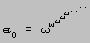( raised to the to the to the , etc., times)

Now we are qualifying over the set of proofs instead of the set of natural numbers. The set of proofs viewed as a finite branching tree has order type 0.

Philosophical Questions

Suppose we admit the provability of the consistency of the formal system for arithmetic. Is that proof epistemologically satisfactory? Is induction through 0 finitary reasoning?

Alonzo Church has a thesis now simply called Church's Thesis. It states that any epistemological proposal put forth as concrete finitary reasoning can be shown to be equivalent to the theory of recursive functions.(54) Gentzen's theory goes beyond the theory of recursive functions. This raises the question: Is Gentzen's proof a part of real mathematics?

Ontological Problem

Hilbert's program still has a division between real and ideal parts of mathematics. What ontological status do objects in the ideal part of mathematics have? They have no reality. They were simply created to give the ideal parts, to give us shortcuts, but never believed to be a part of reality. This gives us a realm of virtual objects, completing the dualism of objects: there are objects that exist, and objects that don't exist.

What is the source of mathematical knowledge? Of mathematical truth?

Paul Bernaceraf raises this dilemma: What is our standard theory of knowledge? Of truth? There is sort of a correspondence theory; knowledge comes to be known because objects impinge upon our cognitive faculties through our senses, and we form beliefs through causal interaction between the object we are thinking about and our thoughts. The formalists and the Platonists have complementary difficulties concerning these matters.

Formalists

According to formalist theory, we have a perfectly reasonable conception of knowledge of objects in real mathematics. With respect to ideal math, we can gain a conception of the objects through the use of a formal system.

However, truths can only be for the real part of mathematics; there are no things corresponding to our beliefs in the ideal part. This results in a dualistic theory of truths-- some thoughts are true through a hybrid, artificial theory, while others are true through normal means.

Platonists

Platonists believed that abstract reality is a reality. Thus, they don't have the problem with truths because objects in the ideal part of mathematics have properties. Instead the Platonists have an epistemological problem-- one can have no knowledge of objects in the ideal part of mathematics; they can't impinge on our senses in any causal way.

This fact, that the formalists have no satisfactory theory of truth while the Platonists have no satisfactory theory of knowledge, is called Bernaceraf's problem. The effect of Benaceraf's paper was to make public the idea that neither the formalists' nor the Platonists' theories were totally acceptable.

Part II: Intuitionism, A Third Direction

II.1 General Introduction to Intuitionism

The intuitionist's picture of mathematics was different. In represented a continuation of the unpopular Kantian approach to mathematics.

Intuitionism was founded in 1907 in the Ph.D. dissertation of L. E. J. Brouwer at the University of Amsterdam. After publishing his dissertation, he introduced several important topological theorems, thus giving him some mainstream mathematical prominence.

Intuitionism embraced two important philosophical theories:

Brouwer's Doctrine-- to be true is to be experienced; whatever exists has its origin in our conscious thought.

Mathematical objects are abstract, a priori, forms of our intuitions.

Two Differences from Kant

Brouwer was a solipsist. He believed the only mind was his own, and was less concerned with intersubjectivity than was Kant.

Brouwer rejected the claim of an a priori intuition of space. Instead, he thought mathematics was based entirely on an a priori intuition of time; Brouwer believed the structure of time guides all conscious activities. The presence of non-Euclidean geometry disallows a single a priori intuition of space.

Brouwer must reconstruct certain parts of mathematics given his own constraints. The positive program of intuitionism is a construction of mathematics as limited by Brouwer's Theory of Consciousness. The negative program of intuitionism argues that standard mathematics is actually wrong (or at least inconsistent).

Brouwer does argue that standard mathematics is inconsistent; his argument is based on his epistemological idealism. Brouwer makes little distinction between Hilbert and the Platonists.

Some of Brouwer's constructions depend on the assumption that if a proposition is true, we can come to know that it is true.

II.2 Intuitionist's Construction of the Natural Numbers

In constructing the natural numbers, there is one fundamental idea: We have the ability to distinguish one thing from another. Then, we must abstract from the difference between the two things, and get the concept of forming an entity with one part and another part. Through this activity, the natural numbers are constructed; the natural number one corresponds to the intuition before performing this mental activity, while two corresponds to the intuition after the activity. Repeating the mental abstraction gives the subsequent natural numbers. It is important to concentrate only on the act itself-- abstracted from the content; one must look at the empty form.

Through this method, the intuitionists also derive the basic numerical operators. An equation: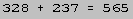is the report of four activities: generating the numbers, looking at two of them together, and recognizing they're the same as a third. Arend Heyting(55) said the role of a math teacher is to make the student carry out the same mental activities as he did.

The point of abstract mathematical notation is to help us generate certain activities. Rules of mathematics abstract away from the actual mental activities. Ultraintuitionism concerns itself with physical limitations as well. Brouwer's standard intuitionism simply limits us to what is finitary.

According to intuitionist theory, reductio ad absurdum proofs are not allowable to prove that something exists (although they are acceptable for negative results.(56)

II.3 Intuitionist's Construction of the Real Numbers

Problem: The previous processes have finite limitations on the number of steps. The set of real numbers requires infinity in some way or another.

Brouwer, in his dissertation (1907), suggested a separate act of consciousness is needed for generating real numbers. He called it ur intuition-- a fundamental idea we all possess corresponding to the continuum. The ur is always growing. By 1918, however, Brouwer had given up the idea of the ur. His general idea was that it is the whole that is important when speaking of the real numbers. The elements of the whole are then abstracted through conditions or limitations. One of the main reasons the idea of ur intuition was discarded was that it lacked any mathematically useful structure.

Real numbers can be seen as a convergent sequence of rational numbers, but sequences are infinite. However, a constructable sequence, with a formula or rule given to generate the elements, is allowable. Thus, the convergent sequence must be capable of being generated by a rule. French mathematicians took the view that the only real numbers that exists are those that are convergent sequences of rationals which are calculable. However, Brouwer noticed the set of calculable algorithms is enumerable (has cardinal number 0). Thus, we can't restrict the real numbers to this set, because it would then not have properties that the uncountable reals have.

Brouwer's solution was a generalization of the notion of an algorithm or rule to give an uncountable number of algorithms to give what is needed for reals. This was the notion of a choice sequence.

II.4 Choice Sequences

Choice sequences represent Brouwer's main contribution to Kantian mathematics.

Ordinarily, an algorithm is a rule for calculating the elements in a sequence. Two things characterize an algorithm: 1) it is rule-like; and 2) it is deterministic (it gives exactly one value). Brouwer generalized algorithms by loosening the requirement that an algorithm be deterministic. The result is a sequence in which an element of a sequence is able to be chosen out of a set of candidates.

A choice sequence is given by a deterministic rule to give the first few elements, and a not-necessarily-deterministic rule for picking subsequent elements. Brouwer pointed out that this corresponds to an a priori intuition of time: the past is fixed, while the future depends on the past, but many possibilities remain.

Example

is a sequence of rationals.

(1) = ½, (2) = ½, (3) = ½, (4) = ½

(n+1) is some rational number such that: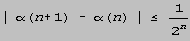Thus (5) is on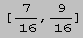, and (6) depends on what is picked for (5).

The canonical choice sequence is the sequence used to generate decimal fractions.

Consider defined exactly as is:

is a sequence of rationals.

(1) = ½, (2) = ½, (3) = ½, (4) = ½

(n+1) is some rational number such that: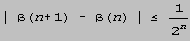Are and the same? Do they converge to the same real number? We don't and cannot know! Some important questions about choice sequences are not answerable in a finite amount of time. Thus, there is no truth concerning questions about the equality of and . We don't even know if we'll know the answer in a finite amount of time.

Brouwer had to rework set theory to coincide with his other constructions. Under his version of set theory, the distinction between an element of a set and the set itself is less well-defined.

The introduction of choice sequences result in contradictions with classical mathematical theorems. For example, there is a classical theorem stating a line has a total order;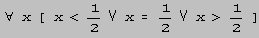This doesn't hold for numbers like ! Thus, order properties of the continuum are weaker in the intuitionist theory.

Brouwer proved a theorem stating that every real-valued function defined on a closed interval is uniformly continuous on that interval. Consider f(x) = 1 for x < ½, f(x) = 3 for x > ½. This function is clearly discontinuous at x = ½. It also appears to be defined over the interval [0,1]. However, in order for Brouwer's theorem to hold, he must show that the function is not defined at some point on the interval. One such point is . We can't tell what f() is equal to. Thus, this is not a counterexample to Brouwer's theorem.

From this, we can see that a function is defined if its value depends on only a finite amount of information about the input. This corresponds identically to continuity.

Two consequences of the property of discontinuity

Brouwer can easily prove the uncountability of the real numbers. Consider a function, f, mapping the reals into the natural numbers. If this is truly a continuous function, its value must be calculable based on a finite amount of information. However, since the natural numbers are discrete, such a function would have to be discontinuous. Let's say f(½) = n. Then, f() = n if = ½, or f() = k, if ½ (where k n). Thus, the function, f, must be undefined at x = . Therefore the function cannot be continuous (it isn't defined everywhere), and the real numbers must be uncountable.(57)

Suppose we want to divide the continuum into two sets, A and B (B = R - A). This activity of forming a subset of the continuum is perfectly natural. Using the method of characteristic functions, we can translate talking about sets into talking about functions. We define fA(x) = 1 if x A, fA(x) = 0 if x A. This method results in undetachable sets-- sets such that they cannot cleanly be picked out of the continuum. The real number may, or may not, be in the set, and the characteristic function for that set is discontinuous. In fact, for any subset of R, the characteristic function for R is discontinuous. In other words, there are no detachable subsets of the continuum. This view of the real number line is the same as Aristotle's. In a sense, we've come full circle, as the problems with the Aristotelian continuum re-appear.

Brouwer noticed that the properties of space thought to be purely geometric can be expressed temporally once we admit that what characterizes the structure of time is that the future is undecided.

The intuitionists and the Platonists agree on one important point: They both believe that the ideal parts of mathematics consist of actual objects created in the mind.

Brouwer, later in his career, admitted that there was a problem with choice sequences. The basic tenet that a real number is created by acts of choice seemed improper-- it required acts of humans, which Brouwer didn't feel should be introduced into mathematics. In the late 1940's, Brouwer introduced the method of the creating subject to generate real numbers. He said we should focus on an idealized mathematician, B, and divide his research into stages. At each stage we ask him the status of an unsolved mathematical problem. We then define the sequence :

(n) = ½ if at the nth stage, B hasn't yet proved or refuted the unsolved problem.

(n) =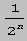if at the nth stage, B has solved the problem.

This process forms a sequence which is a real number; there is no act of choice. Instead, there is an automatic procedure, capturing the same effect as choice sequences, without appealing to the non-mathematical act of choice.

Clearly, this method will not work if the unsolved problem is solved, so, in order for the method of the creating subjects to be an acceptable method, there must be an inexhaustible supply of unsolvable mathematical problems. Brouwer, as a matter of faith, believed this to be true. Hilbert, however, in a famous address to the congress of mathematicians in the late nineteenth century, remarked that there could be no problem which is unsolvable in principle. Brouwer obviously opposed this view.

II.5 General view of Brouwer's Intuitionism vs. Hilbert's Formalism

Both Hilbert and Brouwer were constructivists. Hilbert's Kantianism was very different from Brouwer's, though. Hilbert actually put a structure on the intuitive part of mathematics-- essentially that of finitary thought and formal systems. With Gödel's work, we can see that Hilbert's formal system fits the theory of recursive functions.

Brouwer was very much opposed to these ideas, especially that of formalizing systems. He even opposed the formalization of logic. Brouwer had a very radical view of mathematics and language's relationship. In language, we can communicate the output of mathematical construction, thus helping others recreate the mathematical experience. But, the proof itself--mathematical thought itself--construction itself--is a pre-linguistic, purely conscious activity which is much more flexible than language. Brouwer thought formal systems could never be adequate to cover all the flexible options available to the creative mathematician. Brouwer, in fact, thought formalism was absurd! In particular, Brouwer thought that it was crazy to think that codified logic could capture the rules for correct mathematical thought. He showed particular rules of logic are inadequate. The most famous of these was the law of the excluded middle: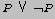fails for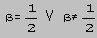. Another such rule, the rule of double negation (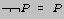) does not hold either. The inadequacy of the rule of double negation is another good reason for rejecting reductio ad absurdum proofs for positive results.

Brouwer hypothesized about the reason why philosophers and mathematicians included the law of the excluded middle. He supposed that logic was codified when the scientific community was concerned only with finite objects. Considering only finite objects, the law of the excluded middle holds. However, a mistake was made when mathematics moved into the infinitary: the rigid rules of logic were maintained without question. Brouwer suggested that no rigid codification should come before the development of mathematics.

A second major distinction between Brouwer and Hilbert was that they disagreed on the position of logic. While Hilbert thought logic was an autonomous, finished science that could be freely applied to other mathematics, Brouwer argued logic should only come after the mathematics is developed.

Brouwer's disciple, Arend Heyting, took on the challenge of explaining to the mathematical community what intutionism is all about. Contrary to Brouwer's wishes, Heyting formalized intuitionistic logic and intuitionistic number theory. Brouwer was furious, but in the end, Heyting's approach won; the intuitionism dealt with today is largely that which Heyting formalized.

Gödel, in the middle 1930s, proved the consistency of classical number theory relative to the consistency of intuitionistic number theory. Gödel, in 1958, gave an even more interesting proof to the effect that one can't proof the consistency of a formal system within a formal system with equivalent finitary limitations. Instead, one must use a less finitary formal system. Intuitionistic number theory is, in fact, less finitary than the formalist's number theory.

Intuitionism's drawbacks

Intuitionistic mathematics is much less familiar, and arguably more complicated than classical mathematical theory.

Many people were unhappy with Brouwer's ontological idealism.(58)

In summary, Brouwer's contribution was more philosophical than it was mathematical. The situation today remains that there is no single philosophy of mathematics that is entirely satisfactory.

Index

a priori 24

Abscissa 21

Abstractness 1

Acute angle hypothesis 14

Analyst, The 22

Anti-Kantian view 23

Applicability 1

Archimidean Postulate 11

Archimides 10

Area of circle 19

Aristotle 5, 50

analysis of infinity 5

logic 5

proof methods 5

Atomic parts 3

Atomism 19

Axiom of choice 38

Axiomatization of Geometry 12

Axioms 6

Babylonians 3, 11

Barrow, Isaac 20

Belttrami 15

Berkeley, George 22

Bernaceraf, Paul 38, 39, 44, 45

Bernaceraf's Problem 45

Betweenness, Hilbert's Postulates 12

Bolyai 14

Bolzano 25

Definition of a limit 25

Brouwer, L. E. J. 46-52

Brouwer's 37

Brouwer's Doctrine 46

Brouwer's Theory of Consciousness 46

Calculus, Invention of 21

Cantor, George 28, 29, 32

Cardinal numbers 2

Category theory 39

Cauchy 25

Definition of a Limit 26

Cauchy's condition for convergence 26

Causal theory of knowledge 39

Cavalieri 17, 19

Cavalieri's principle 17

Characteristic functions, method of 50

Choice sequences 48, 51

Church, Alonzo 44

Church's Thesis 44

Circle, area of 19

Classes 38

Cohen 38

Commensurability 3

Completed infinity 5, 16

Conceptual Foundations of Mathematics 22

Congruence, Hilbert's Postulates 12

Contiguous succession 5

Continuity 3, 28

Aristotle's definition 5

Continuity, Hilbert's Postulates 12

Continuous magnitude 26

Continuum

Intuitionist's theory of 47

Continuum hypothesis 38

Counting 2

Creating subject, method of the 50

Crisis

in the foundation of mathematics 37

Second 22

Third 37

Crisis, First 3

Criterion for convergence

external 26

internal 25

Decimal 2

Dedekind 39

Dedekind, Richard 13, 28, 29, 39

Dedekind Cuts 28

Dedekind's Postulate 13

Deduction 6

Definition of concepts 6

Denumerable set 30

Diagonal Proof 30

Discrete 3

Discrete mathematics 11

Discreteness 4

Doctrine of Logicism 36

Double negation, rule of 51

Egyptians 11

Elements 11

Elements, The 11

Elliptical geometry 15

Empirical intuition 25

Enumerable set 30

Epistemology 1

Epsilon-delta definition of a limit 27

Euclid 6, 11, 16, 33

Elements, The 11

Fifth postulate, his 38

Self-evident assumptions 11

Euclidean geometry 15, 25

Eudoxes 6

Method of Exhaustion 9

Theory of proportion 6

Excluded middle, law of 51

Exhausted 2

Existence postulate 6

Extension 35

External criterion for convergence 26

Fallacious 6

Farmer and the Crow 1

Fifth Postulate of Geometry 12

Figurate numbers 3

Finitary reasoning 40

First-order property 36

fluents 21

fluxions 21

Formal system 16

Formalism 40, 51

Fraenkel, Abraham 41

Frege 28, 34

Function, continuous 26

Galileo

Attack on infinity 17

Criticizing Cavalieri 17

Gauss 14

General Survey 1

Gentzen 43, 44

Genus 6

Geometry

Euclidean 25

Gödel, Curt 44

Gödel, Kurt 38, 39, 42, 43, 51, 52

Completeness Theorem 42

Gödel-number 43

Gödel-numbers 42

Gödel-numbers 42

Greek Reaction to the Downfall 4

Group theory 39

GVB Set Theory 38

Heterogenia 19

Heterogenous indivisible 19

Heyting, Arend 47, 52

Hilbert, David 15, 37, 40, 41, 46, 51, 52

Axiomatization of Geometry 12

Hilbert's Program 40, 43

Hilbert's Foundation of Geometry 12

Hilbert's Postulates

Betweenness 12

Congruence 12

Continuity 12

Incidence 12

Parallelism 12

Hilbert's Program 40

Hilbert's Program 43

Homegenous indivisible 19

Hyperbolic geometry 15

Ideal part of mathematics 40

Incidence, Hilbert's Postulates 12

Inconsistencies 1

Infinite divisibility and discreteness 4

Index set 2

Indirect proof 4

indivisible 17

heterogenous 19

homegenous 19

Indivisibles

vs. infinitesimals 19

Infinite divisibility 3, 4

Infinite divisions 5

Infinite regress 6

Infinitesimal 20

Infinitesimals

vs. indivisibles 19

Infinity 1

Artistotle's analysis 5

attack against 17

Instantiation 6

Integration 18

Internal convergence 27

Internal criterion for convergence 25

Internally inconsistent 4

Intuition 25

Empirical 25

Intuition, pure 25

Intuitionism 37, 46-51

Irrational numbers 3

Isomorphism 5

Kant 46

Kant, Imanual 23, 24

Epistemological Problem 24

First Thesis 24

Klein 15

Kronecker, Leopold 23, 33

Lambert 14

Law of the excluded middle 51

Leibniz 21

Levels of objects 37

Limit 25

Bolzano's Definition 25

Cauchy's Definition 26

Epsilon-delta definition 27

Weierstrass' definition 27

Linguistics 39

Logic 5, 35, 52

Logicism 36

Magnitudes 11

Mathematical induction 33, 44

Metamathematics 41, 42

Method of characteristic functions 50

Method of equilibrium 10

Method of Exhaustion 9-11

Method of fluxions 21

Method of the creating subject 50

Methodology 1

Mill, John Stuart 23

Model 16

Moment of a fluent 21

names 34

Natural Numbers

Intuitionist's construction of 47

Negative program of intuitionism 46

Neutral geometry 14

Newton 21

Newton and Leibniz, Impact of 22

Newton, Isaac 21

Nicholas of Cusa 16

Non-Euclidean geometry 46

discovery 14

evaluation of 13

modeling inside Euclidean geometry 15

Number theory 3

Obtuse angle hypothesis 14

Ontology 1

order-type 32

Ordinal numbers 2

Ordinality

Cantor's notion 32

Ordinates 21

Parallel postulate 12, 14, 38

Negation of 14

Parallelism, Hilbert's Postulates 12

Pascal 20

Peano 33

Pictorial diagrams 11

Plato 4

separation of geometry and number 4

Platonism 35

Positive program of intuitionism 46

Postulates 6

Potential infinity 5

Power set 31

predicates 34

prick of x 21

Principia Mathematica 37, 41, 44

Principle of Comprehension 35

Proclus 13

Proof methods

Aristotle's view 5

Proof, Falsehood of Commensurability 3

Proofs 3

Proper classes 38

Properties

self-referential 36

Property

first-order 36

second-order 36

Pythagoreans 3, 5

Rational 3

Reactions to Berkeley 23

Real Numbers

Intuitionist's Construction of 47

Real part of mathematics 40

Recursive 35

Riemann 15

Rigor 17

Rigorous 17

Russell, Bertrand 34, 36, 37, 41

Saccheri 14

Second Great Crisis 22

Second-order property 36

Self-evident assumptions

11

Self-referential 36

Set 27

Space 24

Spaciotemporal 24

Species 6

spirit of finesse 20

Stevin 17

Straight arrows 50

Structuralism 39

Thales 3

Theory of proportion 6

magnitudes 11

numbers 11

Theory of recursive functions 51

Third Crisis 37

Reactions to 37

Thomas Heath 11

Time 24

Total order 49

True geometry 16

Turing, Alan 42

Type theory 37

Ultraintuitionism 47

Unapplied mathematics 25

Undetachable sets 50

Uninterpreted formal system 16

Unsound proof 9

Ur intuition 48

Valerio 17

Valid 6

Vieta 19

VonNeuman, John 38

Wallis

axiom, his 15

Wallis, John 13, 19, 20

Weierstrass 27

What Are and What Ought to Be the Numbers 39

What is Cantor's Continuum Problem? 39

Zeno 4

Zermelo, Earnst 41

Zobachevsky 14

1. Interestingly, "Calculus" is latin for pebble.

2. In French, the number 80 is said "quatre-vingts" which directly translates as "four twenties."

3. The number 60 has three prime components (2, 3, and 5), one more than either 10 or 20. That additional prime component was useful in calculations.

4. The Pythagoreans were a nearly religious cult who followed Pythagoras and his ideas.

5. One number theoretical discovery the Pythagoreans made is that squares are sums of two successive triangular numbers.

6. Latin for "reduced to an absurdity." Often called an indirect proof or proof by contradiction.

7. Note the way the potential infinity's definition is stated negatively, without mentioning the ultimate existence of a point.

8. A statement generally tells about the relationships of concepts.

9. Going back forever

10. Axioms are self-evident universal truths, while postulates are specific to a particular science, and may be irrelevant to other sciences.

11. From handout "Two Eudoxian Principles."

12. Note that here, plugging into the symbolic interpretation of the theory of proportion, x and y are areas of triangles (the same kind of magnitude) and z and w are line segments (again, same kinds of magnitudes). We couldn't say the area of one triangle relative to its base is equal to the area of the other, relative to its base.

13. The fact that triangles of equal height have areas in proportion to their base had already been proven by the Pythagoras.

14. This Greek idea seems less advanced-- a step backward. The Egyptians and the Babylonians had an integrated view to mathematics.

15. Conversely, given Euclid's fifth postulate, this postulate can be proven.

16. Note that from this it follows that Wallis' axiom is false.

17. This need derives from applications of mathematics to the physical world. Decimal expansion of numbers is one example.

18. Galileo was Cavalieri's teacher.

19. Along with this is the idea of atomism, that all spatial figures are made of atomic parts.

20. This proof uses the homogenous indivisible-- an indivisible with the same number of dimensions as the figure it makes up. This contrasts with the heterogenous indivisible-- one which has fewer dimensions than the whole it sums to.

21. Barrow was Isaac Newton's teacher.

22. There is no justification for this; it is based on the mathematician Pascal who also took the infinitesimal approach. He called it the "spirit of finesse."

23. Also called the "prick of x."

24. This process was called the arithmetization of analysis.

25. Kant was an 18th century German philosopher.

26. Mill was a 19th century American philosopher.

27. Kronecker was a 19th century mathematician.

28. Necessary properties are properties such that it couldn't be otherwise.

29. Pure mathematics is, after all, an a priori science; it involves the study of things known prior to having experience.

30. Mathematics involving the study of space is geometry. That which involves the study of time is arithmetic (the concept of succession). The Calculus, specifically mechanics, studies motion, which is the study of the interaction between space and time.

31. In the case of space, this correct theory is Euclidean Geometry.

32. Readers should be aware of a surprising idiosyncracy in modern day definitions: Cauchy's condition for convergence actually utilizes Balzono's definition.

33. Weierstrass was a German mathematician born in 1815. Unlike many great mathematicians, he made the majority of his contributions later in life, after he was 48 years old.

34. The double bars indicate the cardinality of the set.

35. The symbol means "is a proper subset of." A proper subset is a subset which isn't the whole set.

36. This proof again utilizes the popular method, reductio ad absurdum.

37. The character representing the cardinal number of the natural numbers, 0, is the Hebrew letter alaf subscripted with the Egyptian numeral zero.

38. This remains a question until 1962, at which point the answer is that there is no answer; it is undecidable. Gödel showed the existence of A to be consistent, and Cohen later showed its non-existence to be consistent as well. See Gödel, Escher, and Bach by Douglas Hofstadter for more on undecidable axioms. The hypotheses that 20 = 1 is called the continuum hypothesis.

39. Kronecker was a leading 19th century German mathematician.

40. Interestingly, Cantor had a general nervous breakdown as a result of conflicts with Kronecker. Mathematics today does accept Cantor's work as the foundation of basic mathematical analysis.

41. Peano was an Italian mathematician.

42. The property is said to progress hereditarily upwards.

43. Bertrand Russell commented that "all of mathematics is hostage to these three concepts" pointing to the need for an ontological basis of the three primitive ideas.

44. Russell thought the axioms did, at least, characterize the notion of a progression-- a set of order type . However, sets of order types + * + also fit Peano's axiomatization.

45. Frege was a professor of mathematics at Jena university.

46. Peano actually wrote papers in the artificial language Frege invented.

47. This title was a pun on Dedekind's book, What Are and What Ought to Be the Numbers.

48. David Hilbert was one of the top mathematicians in the twentieth century. No branch of mathematics is without Hilbert's influence, and he was indeed a pioneer of many modern areas of investigation.

49. Hilbert's basic inspiration towards creating formal systems was founded in the consistency proofs for non-Euclidean geometry. Hilbert observed that one can concentrate separately on the language, forgetting about a specific interpretation, thus dealing only with a concrete thing.

50. A formal language is an artificial language designed especially for a branch of mathematics.

51. It is especially interesting to note that David Hilbert's effect on mathematics extended beyond his own direct influence. Hilbert had several famous students, including Zermelo and Fraenkel, who contributed a great deal.

52. Turing was one of the early founders of computer science. He is especially famous for his Turing machine.

53. Here, we're using Cst(S) to mean that the formal system, S, is consistent.

54. Referring to the theory of constructive functions and recursive functions initiated by Curt Gödel.

55. Heyting was a student of Brouwer's.

56. Remember a negative result means showing that something does not exist.

57. Interestingly, Brouwer had a special name for real numbers which were sequences of the same number. They were called straight arrows. Note that the set of all straight arrows is countable.

58. Ironically, Brouwer's epistemological idealism has become quite popular and widespread.